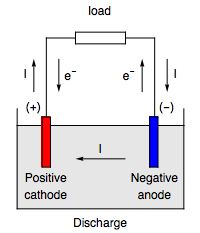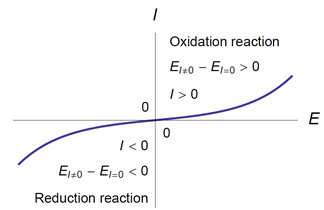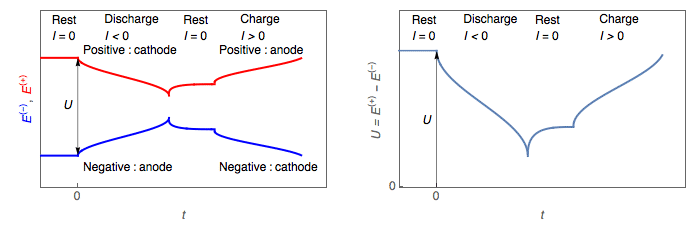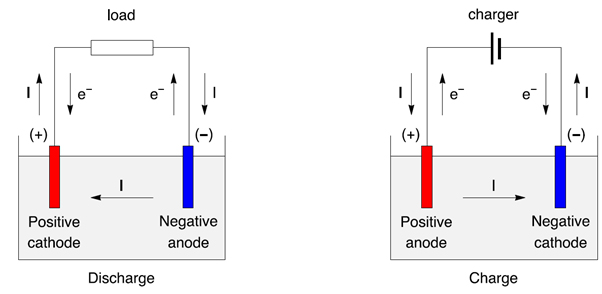Topic 15 min read# Anode vs Cathode: What’s the difference?

Latest updated: June 22, 2023

### Anodes, cathodes, positive and negative electrodes: a definition of terms

Significant developments have been made in the field of rechargeable batteries (sometimes referred to as secondary cells) and much of this work can be attributed to the development of electric vehicles. This work helped lead to the 2019 Nobel Chemistry Prize being awarded for the development of Lithium-Ion batteries. Consequently the terms anode, cathode, positive and negative have all gained increasing visibility.

Articles on new battery electrodes often use the names anode and cathode without specifying whether the battery is discharging or charging. The terms anode, cathode, positive and negative are not synonymous, they can sometimes be confused, which can lead to errors.• An anode is an electrode where an oxidation reaction occurs (loss of electrons for the electroactive species).
• A cathode is an electrode where a reduction reaction occurs (gain of electrons for the electroactive species).
• In a battery, on the same electrode, both reactions can occur, whether the battery is discharging or charging.
• When naming the electrodes, it is better to refer to the positive electrode and the negative electrode.
• The positive electrode is the electrode with a higher potential than the negative electrode.
• During discharge, the positive electrode is a cathode, and the negative electrode is an anode.
• During charge, the positive electrode is an anode, and the negative electrode is a cathode.

### Oxidation and reduction reactions

• An oxidation reaction is an electrochemical reaction that produces electrons. The electrochemical reaction that takes place at the negative of the zinc electrode of a Nickel-Zinc battery during discharge :

$\mathrm{Zn + 4 OH^- \to Zn(OH)_4^{2-} + 2e^-}$

is an oxidation reaction. Oxidation is a loss of electrons.

• A reduction reaction is an electrochemical reaction that consumes electrons. The electrochemical reaction taking place at the positive of a lithium-ion battery during discharge:

$\mathrm{Li_{1-x}CoO_2 + xLi^+ + xe^- \to LiCoO_2}$

is a reduction reaction. Reduction is a gain of electrons.

### Anode, cathode

• An anode is an electrode where an oxidation reaction takes place. The potential of an anode through which a current flows is higher than its equilibrium potential: $E_\text a(I)>E_{I =0}$ (Fig. 1).

• A cathode is an electrode where a reduction reaction takes place. The potential of a cathode through which a current flows is lower than its equilibrium potential: $E_\text c(I)<E_{I=0}$ (Fig. 1).Figure 1: $(E_{I \neq 0}-E_{I= 0})\;I >0$

### Positive and negative electrodes

The two electrodes of a battery or accumulator have different potentials. The electrode with the higher potential is referred to as positive, the electrode with the lower potential is referred to as negative. The electromotive force, emf in V, of the battery is the difference between the potentials of the positive and the negative electrodes when the battery is not working.

### Battery operation

Discharging battery

During the battery discharge, the cell voltage U, I.e the difference between positive and negative, decreases (Figs. 2, 3).

• The positive electrode potential $E_{I \neq 0}^+$ becomes smaller than its value at rest $E_{I=0}^+ : E_{I \neq 0} ^+ < E_{I=0}^+$  → the positive electrode is a cathode.

• The negative electrode potential $E_{I \neq 0}^-$ becomes larger than its value at rest $E_{I=0} ^- : E_{I \neq 0} ^- > E_{I=0}^-$  → the negative electrode is an anode.Figure 2: Discharge and charge of a battery: left, potential change of the positive and negative electrodes; right, battery voltage change

#### Battery charging

During a battery charge, the cell voltage U, the difference between positive and negative, increases (Figs. 2, 3).

• The positive electrode potential $E_{I \neq 0} ^+$ becomes larger than its value at rest $E_{I=0} ^+ : E_{I \neq 0}^+ > E_{I=0}^+$ → the positive electrode is an anode.

• The negative electrode potential $E_{I \neq 0}^-$ becomes smaller than its value at rest $E_{I=0}^- : E_{I \neq 0}^- < E_{I=0}^-$ → the negative electrode is a cathode.Figure 3: Discharge/charge of a secondary battery represented as an electrochemical cell, with electrons and current direction.

### Conclusion

During normal use of a rechargeable battery, the potential of the positive electrode, in both discharge and recharge, remains greater than the potential of the negative electrode. On the other hand, the role of each electrode is switched during the discharge/charge cycle.

• During discharge the positive is a cathode, the negative is an anode.
• During charge the positive is an anode, the negative is a cathode.

Texts describing battery anodes or cathodes certainly implicitly consider the case of the discharge. Let us not hesitate to write, paraphrasing Rutherford, implicit is nothing but poor explicit.

### Find out more about potentiostats and battery cyclers

To find out more about BioLogic potentiostats which are used in battery research and battery testing among other research fields of interest please click our potentiostat overview page and Battery Testing/Battery Cycling overview page.

### Find out more about Battery Cycling Curves

The following article on “How to read battery cycling curves” may be of interest

battery anode cathode positive negative electrode